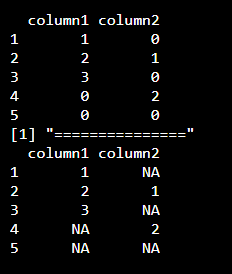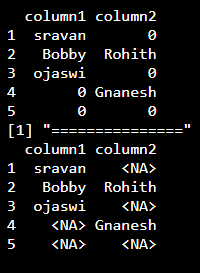Open in App
Not now

# Replace 0 with NA in R DataFrame

• Last Updated : 31 Aug, 2021

In this article, we are going to discuss how to replace 0 with NA values in dataframe in R Programming Language.

NA stands for Null values which can represent Null data / Null elements in a dataframe. The task can be achieved by first defining a dataframe that contains 0 as values. Then we can replace 0 with NA by using index operator [].

Syntax:

dataframe[dataframe== 0] = NA

where,

• dataframe is the input dataframe
• In index we are checking if the value is 0, if it is 0 then we are replacing it as NA

Example: Replacing 0 with NA for integer data

## R

 `# create a dataframe with 0 ``# along with numeric values and display``data=``data.frame``(column1=``c``(1,2,3,0,0),``                ``column2=``c``(0,1,0,2,0))` `# Actual data``data` `# replace 0 with NA``data[data==0]=``NA` `print``(``"==============="``)` `# display``data`

Output:Example: R program to create a dataframe with strings along with 0 and replace 0 with NA

## R

 `# create a dataframe with 0 ``# along with string values and display``data=``data.frame``(column1=``c``(``"sravan"``,``"Bobby"``,``"ojaswi"``,0,0),``                ``column2=``c``(0,``"Rohith"``,0,``"Gnanesh"``,0))` `# Actual data``data` `# replace 0 with NA``data[data==0]=``NA` `print``(``"==============="``)` `# display``data`

Output:My Personal Notes arrow_drop_up